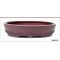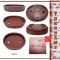##### The Japanese Bonsai specialist
Direct order Contact Help / Services Newsletter# Tokoname riveted brown pot 106f12

› Bonsai pots › Tokoname bonsai potsref. : 5014

114,50

Available quantity : 2Order

###### Description

Exterior: 160*125 mm. Height: 34 mm. Interior: 142*110 mm. Height: 24 mm. An exceptional piece of Tokoname ware with raised dots and an unglazed, matt natural look. The red-brown colour of this bonsai pot comes from the fine-grained clay containing iron, which is typical of the area. The city of Tokoname has been a centre for pottery for thousands of years.

#tokoname 6.8 #bonsai 3.6 #brown 3.5 #riveted 2.7 #height 2.6 #pots 2.4 #exceptional 2.1 #containing 2 #thousands 1.9 #unglazed 1.8

Formule
(( ROUND((CHAR_LENGTH(b.article_nom)-CHAR_LENGTH(REPLACE(b.article_nom, 'tokoname', '')))/LENGTH('tokoname')) + ROUND((CHAR_LENGTH(b.article_description)-CHAR_LENGTH(REPLACE(b.article_description, 'tokoname', '')))/LENGTH('tokoname')) ) * 4.8) + (( ROUND((CHAR_LENGTH(b.article_nom)-CHAR_LENGTH(REPLACE(b.article_nom, 'brown', '')))/LENGTH('brown')) + ROUND((CHAR_LENGTH(b.article_description)-CHAR_LENGTH(REPLACE(b.article_description, 'brown', '')))/LENGTH('brown')) ) * 3.5) + (( ROUND((CHAR_LENGTH(b.article_nom)-CHAR_LENGTH(REPLACE(b.article_nom, 'riveted', '')))/LENGTH('riveted')) + ROUND((CHAR_LENGTH(b.article_description)-CHAR_LENGTH(REPLACE(b.article_description, 'riveted', '')))/LENGTH('riveted')) ) * 2.7) + (( ROUND((CHAR_LENGTH(b.article_nom)-CHAR_LENGTH(REPLACE(b.article_nom, 'height', '')))/LENGTH('height')) + ROUND((CHAR_LENGTH(b.article_description)-CHAR_LENGTH(REPLACE(b.article_description, 'height', '')))/LENGTH('height')) ) * 2.6) + (( ROUND((CHAR_LENGTH(b.article_nom)-CHAR_LENGTH(REPLACE(b.article_nom, 'exceptional', '')))/LENGTH('exceptional')) + ROUND((CHAR_LENGTH(b.article_description)-CHAR_LENGTH(REPLACE(b.article_description, 'exceptional', '')))/LENGTH('exceptional')) ) * 2.1) + (( ROUND((CHAR_LENGTH(b.article_nom)-CHAR_LENGTH(REPLACE(b.article_nom, 'containing', '')))/LENGTH('containing')) + ROUND((CHAR_LENGTH(b.article_description)-CHAR_LENGTH(REPLACE(b.article_description, 'containing', '')))/LENGTH('containing')) ) * 2) + (( ROUND((CHAR_LENGTH(b.article_nom)-CHAR_LENGTH(REPLACE(b.article_nom, 'thousands', '')))/LENGTH('thousands')) + ROUND((CHAR_LENGTH(b.article_description)-CHAR_LENGTH(REPLACE(b.article_description, 'thousands', '')))/LENGTH('thousands')) ) * 1.9) + (( ROUND((CHAR_LENGTH(b.article_nom)-CHAR_LENGTH(REPLACE(b.article_nom, 'exterior', '')))/LENGTH('exterior')) + ROUND((CHAR_LENGTH(b.article_description)-CHAR_LENGTH(REPLACE(b.article_description, 'exterior', '')))/LENGTH('exterior')) ) * 1.8) + (( ROUND((CHAR_LENGTH(b.article_nom)-CHAR_LENGTH(REPLACE(b.article_nom, 'interior', '')))/LENGTH('interior')) + ROUND((CHAR_LENGTH(b.article_description)-CHAR_LENGTH(REPLACE(b.article_description, 'interior', '')))/LENGTH('interior')) ) * 1.8) + (( ROUND((CHAR_LENGTH(b.article_nom)-CHAR_LENGTH(REPLACE(b.article_nom, 'unglazed', '')))/LENGTH('unglazed')) + ROUND((CHAR_LENGTH(b.article_description)-CHAR_LENGTH(REPLACE(b.article_description, 'unglazed', '')))/LENGTH('unglazed')) ) * 1.8)

## Secure payment## Delivery

Our logistic partners :04 74 55 23 48
Pépinière MAILLOT-BONSAÏ
Le Bois Frazy
01990 RELEVANT - FRANCE
on appointmentThe Japanese Bonsai specialist
If you like Maillot Bonsaï, share on  facebook

A vos côtés. Les services restent les mêmes.

La vente continue et les expéditions également.

Meilleurs vœux pour 2021.

Guy Maillot

OK